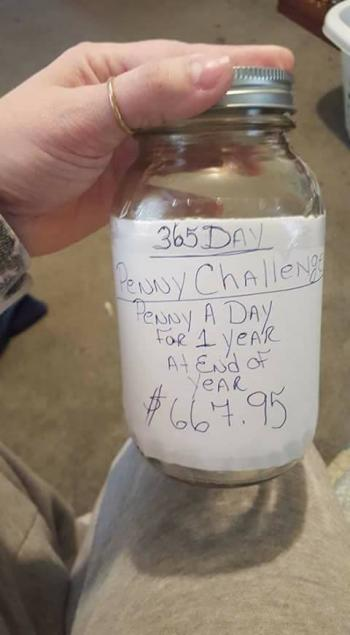Games
Problems
Go Pro!Since it's almost the new year, this image (click the image for a larger view) is making the rounds on facebook again, and everyone is reading it and saying, "WHAT? How does that work out to \$667.95?"

The answer is, it doesn't. It works out to exactly what you thought it would work out to: 365 pennies, or \$3.65. Unless it was Leap Year, and then it would work out to \$3.66. Not terribly hard math.

However, even though "a penny a day" is what they said, "a penny a day" is not what they meant. How do I know that? I'll show you in just a minute.

What they meant was, on January 1st you put in one penny. On January 2nd, you put in two pennies. On the 3rd, three pennies. And so on, until December 31st, when you put in 365 pennies, because it's the 365th day of the year.

How do I know that's what they meant? Because there's a really nice formula to calculate the sum of the first n integers:

Sum = n(n + 1)/2

In this case, n = 365, so the sum is:

Sum = 365(365 + 1)/2 = 66,795.

And that's how I know that's what they meant - because if that's what they meant, the math works out correctly to \$667.95.

And by the way...if it was leap year?

Sum = 366(366 + 1)/2 = 67,161, or \$671.61.

So yes, this will work out to save you a pretty good sum of money by the end of the year. Maybe enough to pay a month's rent, depending on where you live!

Of course, it's not as good as doubling the number of pennies each day; that would save you a boatload of money over the course of a year:

1 + 2 + 4 + ... + 2n = 2n + 1 - 1

So: 1 + 2 + 4 + ... + 2365 = 2366  - 1 = 1.5 x 10110.*

Actually, scratch my last comment; that's not a boatload of money. If a penny's volume is 0.35 cm3, or 0.00000035 m3, that's a volume of 5.25 x 10103 m3. Considering the volume of the sun is 1.4 x 1027 m3, I don't think you're going to fit those pennies in a jar, a boat, or even all the planets of the solar system. Maybe we should just stick to the original plan.

Incidentally, there's also a "Dollar a Week Challenge" in which you save one dollar the first week, two dollars the second week, and so on for an entire year. It sounds like a lot more, since you're saving a dollar instead of a penny, but there are only 52 weeks in the year, so it works out like this:

Sum = 52(52 + 1)/2 = \$1,378, which is only a litte more than twice the penny challenge.

If you wanted to get really interesting, you could do a "Dollar Square Weekly Challenge," which would look something like this:

In the first week, you save 12 = 1 dollar.
In the second week, you save 22 = 4 dollars.

And so forth. It turns out, there's a nice formula for the sum of squares as well:

Sum = n(n + 1)(2n + 1)/6

Sum = 52(52 + 1)(2 x 52 + 1)/6 = \$48,230. That's a lot of money! How would it compare to a Penny Square Daily Challenge?

Sum = 365(365 + 1)(2 x 365 + 1)/6 = 16,275,715 pennies, or \$162,757.15. So even though the Dollar Challenge saves more than the Penny Challenge, the Penny Square Challenge saves more than the Dollar Square Challenge.

It would be interesting to see how many weeks you'd have to do the Dollar Square Challenge in order for it to surpass the Penny Square Challenge for a year. I'll leave that do the reader to figure out!

* This formula is actually for a leap year. Since the first term of the sequence is 20, the 366th term is 2365.

# Blogs on This SiteReviews and book lists - books we love!The site administrator fields questions from visitors.Like us on Facebook to get updates about new resources